Arabi, M., and  Dahmardeh Ghalehno, M. (2023). "Suitability of thin-layer drying models for halogen lamp drying of sugarcane bagasse," BioResources 18(1), 1052-1071.

#### Abstract

The drying process of bagasse particles was investigated in this study using an artificial neural network (ANN) and 18 thin-layer drying (TLD) models. These models are used for investigating kinetics and understanding engineering parameters involved in the drying process of food and agricultural products. Bagasse particles were studied at 105 to 135 °C and an initial moisture content of 180% (based on the dry weight) using a halogen moisture analyzer. The results showed that an increase in the temperature decreased the bagasse drying period and increased the constant drying. The whole drying process of bagasse happened in a falling drying rate period. The fitness of drying curves on semi-experimental TLD models based on statistical parameters, including root mean square error (RMSE), sum of square errors (SSE), and coefficient of determination (R2) showed that the Hii et al. model had the highest coefficient of determination and the lowest error percentage. The ANN predicted changes in the bagasse moisture content through time more accurately than the Hii et al model. Also, the results demonstrated that the selected ANN model and a number of semi-empirical models with less than 3 adjustable parameters provided good agreement and can be considered promising tools to predict drying kinetic of bagasse particles.

Suitability of Thin- Layer Drying Models for Halogen Lamp Drying of Sugarcane Bagasse

The drying process of bagasse particles was investigated in this study using an artificial neural network (ANN) and 18 thin-layer drying (TLD) models. These models are used for investigating kinetics and understanding engineering parameters involved in the drying process of food and agricultural products. Bagasse particles were studied at 105 to 135 °C and an initial moisture content of 180% (based on the dry weight) using a halogen moisture analyzer. The results showed that an increase in the temperature decreased the bagasse drying period and increased the constant drying. The whole drying process of bagasse happened in a falling drying rate period. The fitness of drying curves on semi-experimental TLD models based on statistical parameters, including root mean square error (RMSE), sum of square errors (SSE), and coefficient of determination (R2) showed that the Hii et al. model had the highest coefficient of determination and the lowest error percentage. The ANN predicted changes in the bagasse moisture content through time more accurately than the Hii et al model. Also, the results demonstrated that the selected ANN model and a number of semi-empirical models with less than 3 adjustable parameters provided good agreement and can be considered promising tools to predict drying kinetic of bagasse particles.

DOI: 10.15376/biores.18.1.1052-1071

Keywords: Bagasse; Halogen moisture analyzer; Drying kinetics; Artificial neural network

Contact information: Department of Wood Science and Technology, Faculty of Natural Resources, University of Zabol, Zabol, Iran; *Corresponding author: marabi@uoz.ac.ir

INTRODUCTION

Bagasse is essentially the fiber-based residue of the sugarcane’s stalk after the process of syrup extraction. Bagasse is a renewable, sustainable, eco-friendly, bio-degradable, and cheap raw material that is used very frequently on the industrial scale. For instance, bagasse is used as a fiber and as biofuel in the papermaking industries, and it is used to manufacture sugar, bio-alcohol, yeast, animal feed, and wood composites such as MDF and particleboard (Martinez-He rnandez et al. 2018). In Iran, sugarcane can only be cultivated and exploited in Khuzestan province. Historical evidence indicates that sugarcane cultivation in this province dates back to 2000 years ago. The name of this province means the sugar region, which denotes the great importance of sugarcane cultivation and sugar production to the economy of this region in the past. Iranian sugarcane production facilities annually produce nearly 1.2 million tons of excess bagasse, which is burned due to the lack of conversion industries. Burning and eliminating bagasse cause greenhouse gas emission, which contributes to climate change. Burning agricultural residues directly in the field is largely responsible for the production of CO2 (40%), CO (32%), and dust (20%) in the earth’s atmosphere (Kambis and Leivne 1996; Ravindra et al. 2019). In addition to emitting pollutant gases and causing drastic environmental impacts, burning damp agricultural residue impoverishes agricultural fields as it depletes carbon molecules (which should be stored in the soil) by sending them back to the atmosphere (Lemieux et al. 2004; Zhang et al. 2011; Adejumo and Adebiyi 2020). Additionally, burning bagasse is a threat to human health, as a higher occurrence of asthma attack is correlated with the burning time of bagasse (Boopathy et al. 2002).

Fortunately, bagasse can be used as a lignocellulosic feedstock in various industries, which avoids the overexploitation of other nonrenewable lignocellulosic sources. The industrialized use of bagasse is negatively impacted by high moisture content; the processed bagasse contains 50% moisture (based on wet weight). Moisture compromises the industrialized use of bagasse and reduces both the bagasse burning rate and energy production efficiency, which are the main obstacles to the application of wet bagasse as the energy supplier in sugarcane manufacturing (Bahadori et al. 2012). A 3 to 4% decrease in the bagasse moisture content increases the gross calorific value and boiler efficiency by 700 to 800 KJ/kg and 1.5 to 2%, respectively (Sudhakaran and Vijay 2013). This is because the moisture content of bagasse particles must be reduced to 8% before they are used in the production of wood composites such as particleboard and MDF (Pang 2000). A high level of moisture in the bagasse passing through the dryer increases the vapor pressure in the board thickness and bowing of the board after the pressing process. Therefore, it is essential to control the final moisture of processed raw materials from the dryer, but this is time-consuming and problematic in traditional methods such as using an oven. The use of halogen dryers is a rapid method for determining the percentage of moisture in the production lines of different industries and controlling the final moisture content of processed materials (Raj and Stalin 2016; Sicoe et al. 2018).

Although hot air dryers are most commonly used for drying agricultural residue and lignocellulosic materials, their efficiency in the falling rate period of drying is lower than radiant dryers such as halogen lamps. This is because the temperature is directly (without any contact with the surrounding air) brought to the surface of materials under drying, resulting in an increase in the rate of heat transfer to the surface of solid materials (Sharma and Prasad 2001; Al‐Hilphy et al. 2021; Huang et al. 2021). Du et al (2005) and Huang et al. (2021) studied the drying process of wood strands and lignocellulosic biomass by microwave heating dryers and concluded that these dryers functioned more effectively than hot air dryers at moisture contents below the fiber saturation point (FSP) point.

Drying of materials is a complicated process because mass and temperature are simultaneously transferred inside the material and its surrounding environment (Midilli et al. 2002; Erbay and Icier 2009; Ghazanfari et al. 2016; Ertekin and Firat 2017). Drying lignocellulosic biomass is also a complex, dynamic, highly nonlinear, and energy-intensive process involving simultaneous heat and mass transfer (Ribeiro et al. 2019; Han et al. 2020; EL-Mesery et al. 2022). The theoretical modeling of lignocellulosic biomass drying is very complicated because lignocellulosic biomass fiber shrinks anisotropically during the drying process (Sander et al. 2001; Soni 2007). It seems that semi-empirical thin-layer drying models developed on a laboratory scale are a suitable option for examining the drying kinetics of biomass materials (Babalis et al. 2006; Demir et al. 2007; Doymaz 2007a; Hii et al. 2009). Thin-layer drying (TLD) models and curves, which are known as specific curves of the drying rate, have been used to characterize agricultural residues and their drying kinetics (Verma et al. 1985; Kaleta and Górnicki 2010; Gan and Poh 2014;; Buzrul 2022).

The models and curves of TLD and convective dryers have been used to study the drying kinetics of food materials (Wang and Singh 1978; Vega et al. 2007; Kumar et al. 2012), agricultural products (Lee and Kim 2009; Kaleta et al. 2013; Kaur and Singh 2014), lignocellulosic biomass (Zarea Hosseinabadi et al. 2012; Arabi et al. 2017; Brys et al. 2021), and sugarcane bagasse (Freire et al. 2001; Vijayaraj and Saravanan 2007; Shah and Joshi 2010). However, the effect of halogen rays has not yet been studied on bagasse particle drying kinetics. Halogen dryers represent an innovative, fast, and very accurate method for determining the moisture content in a wide range of materials (Kambis and Leivne 1996; Ravindra et al. 2019). For example, the ASTM D6980 (2012) standard describes a standard test for determining moisture content and drying plastic granules using a halogen moisture analyzer. This is the first study to investigate the sugarcane bagasse drying process with halogen lamps. The drying process of agricultural and lignocellulosic materials usually takes place in three different periods, including constant drying rate, the first falling rate period of drying, and the second falling rate period of drying (Ghazanfari et al. 2006). Low energy efficiency and lengthy drying periods are important disadvantages of hot-air drying. In hot-air dryers, the heat transfer to the inner parts of materials under drying is limited, and energy efficiency is low owing to low thermal conductivity, leading to longer periods to dry materials (Du et al. 2005; Huang et al. 2016). In this study, the bagasse particles’ drying process with halogen rays was investigated to solve the mentioned problem, avoid low thermal conductivity and energy inefficiency, and obtain an effective thermal process. In addition to TLD models, the neural network (NN) is one of the latest modeling techniques and predicts complicated physical processes that can predict materials’ drying kinetics very accurately. The NN has been used for modeling the drying kinetics of agricultural products and the drying process of wood (Celma et al. 2008; Dash et al. 2020; Saxena et al. 2022). The results of previous studies clearly show that the ANN predicts the drying process of materials more effectively than TLD models (Chai et al. 2019; Sadeghi et al. 2019).

Therefore, the objectives of this study were to study bagasse drying kinetics and the effect of temperature on the process of bagasse drying using a halogen lamp dryer; to fit bagasse drying curves on TLD models; to determine the most appropriate model; and to predict the bagasse moisture content over time using an artificial neural network (ANN).

EXPERIMENTAL

Materials

Bagasse particles used in this study were obtained from the Neopan Karon Company. The dimension of bagasse particles were 8 to 13 mm length and 0.5 to 2 mm thickness. Its density was 0.12 g/cm3. Bagasse drying experiments were conducted with an initial moisture content of 180% (based on the dry weight) to study the drying rate of bagasse particles under a moisture content higher than the FSP. Therefore, bagasse particles were mixed with water (with the equivalent weight ratio) for 24 h. The samples were kept in sealed polyethylene bags at 3 to 5 °C for 7 days so that the moisture was distributed evenly. During this period, samples were mixed daily to maintain homogenous moisture content in all bagasse particles.

Drying Process

The drying process of bagasse particles was conducted using a halogen moisture analyzer (Model MB45, OHAUS Company, University of Tehran, Iran) at three temperatures of 105, 120, and 135 °C. The MB45 has a sample capacity of 45 g, with a readability of 0.001 g and repeatability to 0.015% (using a 10 g sample). It is shown in Fig. 1. The heater is a halogen lamp, and the operating temperature range is 50 to 200 ºC in 1º increments. During the drying operation, the moisture content was constantly calculated based on the gravimetric method, and the weight difference between before and after drying to represent the loss of moisture. This drying method is called loss-on-drying (LOD), which is a standard method for measuring moisture. The sample is weighed, receives heat, is dried, and then is weighed again. This device is used for testing moisture and analyzing the moisture content in samples such as wood, pulp, biomass, granules, edible materials, food products, and pastes. A series of tasks were followed to study the drying kinetics of bagasse particles. First, 4 g of bagasse particles was evenly distributed on the surface of a stainless-steel tray for keeping samples inside a halogen dryer chamber. The surface of the tray was covered with an identical thickness of bagasse particles. A decrease in the samples’ moisture content at 105, 120, and 130 °C was recorded every 30 seconds to study the effect of temperature on the drying rate of bagasse particles. All experiments were repeated in triplicate for each temperature, and the average values of the moisture content for each temperature were used for drawing and fitting drying curves.Fig. 1. Halogen moisture Analyzer, MB45 AM (ohaus.com)

Drying Kinetics

Drying is one of the widely used methods for the preservation of cereals, fruits, and vegetables. These models are used for estimating the time in which several products are dried under various drying conditions, improving the efficiency of the drying process, and generalizing drying curves for the design and function of dryers. Equation 1 is used for determining the moisture ratio (MR) of bagasse particles,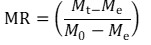(1)

where MR is the moisture ratio (dimensionless), Mt is the momentary moisture content per kg of solid matter/kg of water, Me is equilibrium moisture (kg of material/kg of water), and M0 is the initial moisture content (kg of material/kg of water), where their values are expressed on a dry basis. In the IR process, samples may be dried as much as dry solid content (Celma et al. 2008). Especially for long drying times, Me is relatively small in comparison with Mt or M0. Therefore, Therefore, Eq. 1 is simplified as Eq. 2 (Shah and Josh 2010; Doymaz et al. 2007).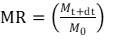(2)

The evaporation ratio in bagasse particles was calculated using Eq. 3,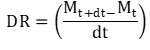(3)

where DR is the drying rate (kg/s), Mt + dt is the moisture content (kg water/kg d.b) at the time t + dt, Mt is the moisture content in the time t, and t is the drying time (s). To determine the MR in this study, experimental data were examined for 18 most important and commonly used models that are used to predict the MR of food and other agricultural products (Table 1). The fixed variables and the constant drying of each TLD model were analyzed and obtained based on the time (the independent variable) using MATLAB 2016 software. The best model was determined using three statistical parameters, namely coefficient of determination (R2), root mean square error (RMSE), and the sum of squared errors (SSE). The fit of a model with the highest value for R2 and lowest values for SSE and RMSE best describes the drying characteristics of bagasse particles. The values for R2, RMSE, and SSE were calculated using Eqs. 4, 5, and 6, respectively,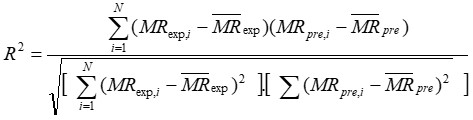(4)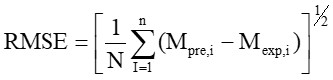(5)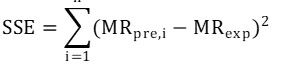(6)

where Mexpi and Mpre,i are respectively the observed and model-predicted bagasse particles moisture contents in the ith measurement, , N is the number of observations (data), and n is the number of constants used in the equation.

Artificial Neural Network Model

The multilayer perceptron (MLP) is a widely used and practical ANN model that was chosen from the ANN toolbox of MATLAB v.16 to predict the MR. In this model, the drying time and temperature of bagasse particles and MR were introduced as the input and output data, respectively. To acquire a suitable topology of the ANN, it is trained with experimental data, and the best topology was determined by trial and error and minimizing the ANN error (Sadeghi et al. 2019; Haftkhani et al. 2021).

The mathematical equation of the MLP ANN is as follows,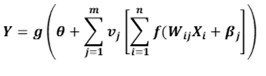(7)

where Y is the dependent variable, g (.) is the activation function of output neurons, θ is the bias value for output neurons, vj is the value of weights between the jth output neuron and hidden neurons, f (.) is the activation function of hidden neurons, Wij is the weights between the jth input neuron and the jth hidden neuron, Xi is the input value of the ith dependent variable, and βj is the jth hidden neuron bias.

Table 1. Mathematical Models Applied to Drying Curves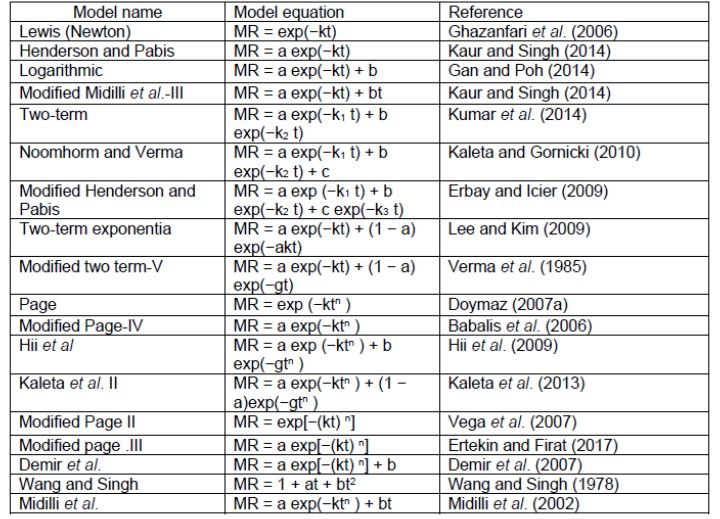In this study, 70, 15, and 15% of the data were randomly selected for training, validation, and testing purposes, respectively. The Levenberg-Marquardt algorithm was chosen for training data. The activation function of the output neuron was linear, but that of the hidden neuron was a hyperbolic-tangent-sigmoid function as follows,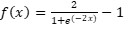(8)

where f(x) and x are neurons’ output and input values, respectively. The structure of MLP for predicting MR is shown in Fig. 2. Also, it was reported in earlier works (Sadeghi et al. 2019; Haftkhani et al. 2021) that a network with one hidden layer and hyperbolic tangent sigmoid (tansig) function is commonly used for forecasting in practice. The statistical parameters MSE, MAPE, and R2 were used for assessing the efficiency of the ANN model to investigate the error value and match the real and predicted data. These statistical parameters were calculated using Eqs. 9, 10, and 11,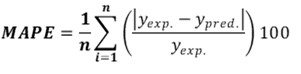(9)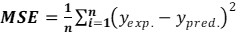(10)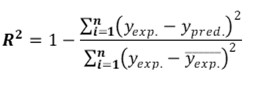(11)

where yexp and ypred are the real and predicted data, respectively, is the average of real data, and n is the number of data.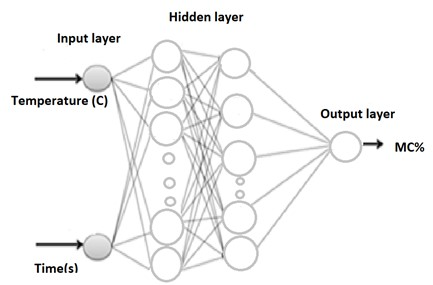Fig. 2. Schema of artificial neural network for prediction moisture content of bagasse particle

RESULTS AND DISCUSSION

To change the anatomy and position of moisture in hygroscopic materials, the temperature is used both directly and indirectly during the drying process. Changes in the bagasse MR in different temperatures over time, influenced by the direct heat transfer, on the surface of bagasse particles are shown in Fig. 3, indicating an exponential reduction in the bagasse MR during the drying process. The bagasse particle MR at 105, 120, and 135 °C approached zero in 1110, 630, and 420 seconds, respectively. The change in the moisture content of bagasse particles was significantly influenced by increasing the temperature from 105 to 135 °C (an increase of 30 °C), which reduced the bagasse drying time (reducing the bagasse moist content) by 2.7 times (Fig. 3).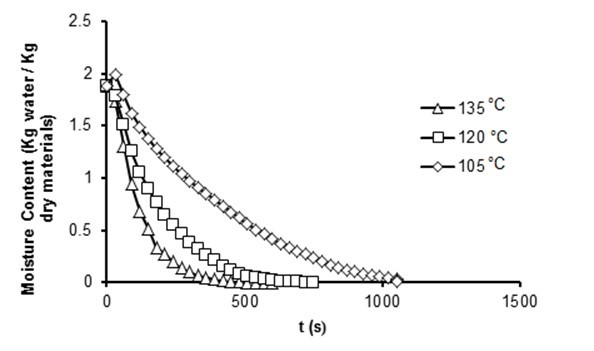Fig. 3. Moisture content versus drying time at different halogen drying temperature

Drying with halogen radiation involves heating with electromagnetic radiation having a wavelength ranging from 0.76 to 2.5 μm. Halogen radiant energy can be easily passed through the air without heating the ambient air. When the halogen lamp is radiated toward the cellulosic materials, in general, reflection varies within the range between 10 and 30%, and the penetration depth of the radiation is close to 0.3 mm. This means that the cellulosic materials surface absorbs 70 to 90% of all incident IR radiation. So, the surface of cellulosic materials becomes strongly heated and then a thermal gradient within the material significantly increases in a short period by conduction. In most previous studies, the impact of temperature on the bagasse drying process was studied indirectly using hot-air dryers. Recently, the direct radiation of IR and microwave dryer has been used in a few studies, indicating a reduction in the drying period using infrared radiation compared with the hot air dryers, especially in the falling drying rate period (Huang et al. 2021). Infrared energy penetrates into the materials and then is converted to heat without heating the adjacent air. Thus, the internal part of materials would be warmer than the surrounding air and the rate of heat transfer is greater as compared to the hot air drying technique (Huang et al. 2021; Al‐Hilphy et al. 2021). Figure 4 shows the bagasse drying rate against its moisture content. Accordingly, the slope of drying curves changes during the drying period of bagasse particles. Depending on the physical features and the moisture content of the materials, the drying process of hygroscopic materials takes place in three separate stages, including the constant drying rate and the first and the second falling rate period of drying. According to Fig. 5, a constant drying rate was not observed in any of the drying temperatures of bagasse particles, and the whole drying process of bagasse particles happened during the falling rate period of drying. Vijayaraj et al. (2007) dried bagasse particles in at 80 to 120 °C and an air velocity of 0.5 to 2 m/sec. Their results showed that the entire drying process happened in the falling rate period of drying despite a high initial moisture content of bagasse. Freire et al. (2001) and Shah and Joshi (2010) observed no constant drying rate in the drying process of bagasse. Du et al. (2005) investigated the drying process of wood chips in convective and microwave dryer. Their results showed that the value for the output moisture microwave dryer was approximately twice that of convective dryers in the initial 100 seconds.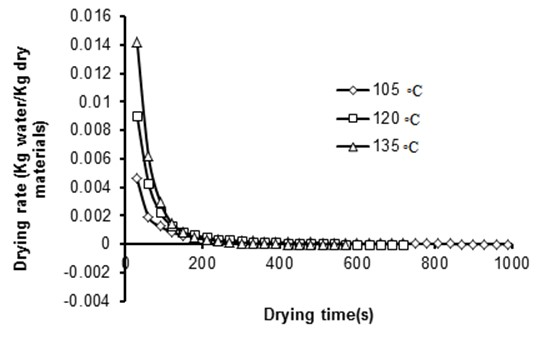Fig. 4. Drying rate versus drying time at different halogen drying temperature

When the moisture of the surface layer in the sample evaporates very quickly, the surface of particles dries very quickly with shrinkage, which reduces the diameter of cellular pores and cavities. Therefore, quick transfer and moisture extraction from the lower layers close to the surface of particles are not possible when there is free water in the lower layers of the surface layer. In fact, the moisture evaporation rate from the surface of the particles is faster than the surface layer’s rate of feeding moisture from its lower layers. Therefore, it is not possible to observe a constant drying rate. In this study, using the halogen lamp and direct transfer of the temperature to bagasse particles caused a fast reduction of the water-air interface and its fast recess to the pores near the surface. As a result, it was difficult to observe a constant drying rate. This type of drying behavior can also be interpreted based on the Biot (Bi) number. For solid materials with a Bi number less than 0.1, the process of drying takes place at a constant rate (Sander et al. 2001), while the Bi number for lignocellulosic materials has been reported to be more than 0.2 (Soni 2007). The first (A-B) and second (B-C) falling rate periods of drying are obvious in the curves of the bagasse drying rate, (Fig. 5). In the first falling rate period of drying, the moisture moves from lower layers from the wood surface to its outer surface (from higher to lower concentrations), which is influenced by the diffusion mechanism. At the beginning of this period, wood surface lower layers are rich in moisture and the drying rate of bagasse particles is high, with a steeper slope of particle drying curves. The moisture content of particles’ lower layers is reduced over time, making the moisture exhaust from bagasse particles more difficult. This increases the surface temperature of the particles, and a temperature gradient is formed contrary to the direction of the moisture gradient in the particles. In lower moisture contents, a sharp deviation is observed in the slope of the drying curve, suggesting that there is no free water available in this stage of drying, with a completely dry surface of fibers. Therefore, this minimizes the drying rate of particles and increases the drying period. This period of drying is known as the second falling rate period of drying.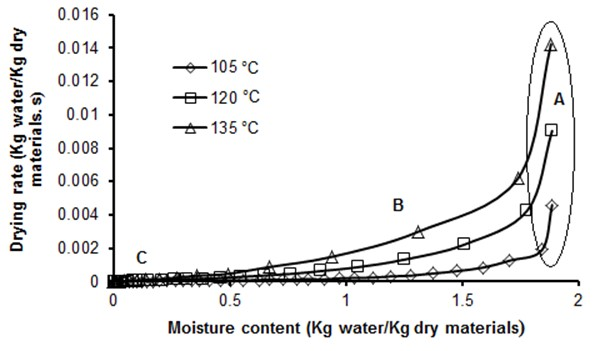Fig. 5. Drying rate versus moisture content at different halogen drying temperature

Drying Curves Fitting

Modeling the drying process of agricultural products is based on a collection of accurate and simple mathematical equations that can adequately describe various conditions of the drying process and drying materials. Eighteen models (Table 1) were fitted on the drying curves obtained from the experimental data using MATLAB 2016 software. The best TLD model was selected based on a higher value of R2 and lower values of SSE and RMSE. The results from the fit of mathematical models on the experimental data showed that the Hii et al. model had the best fit on the experimental data. The Hii et al. model is a combination of the Page and two-term drying model (Hii et al. 2009).

The results from the fit of mathematical models on the experimental data with their fixed coefficients and the values for statistical parameters at different temperatures are shown in Tables 2, 3, and 4. A careful inspection of these results revealed that there was only a limited difference between the results of Hii et al. model as best model and other TLD models. According to Tables 2, 3, and 4, most of TLD models showed relatively high values of R2 (0.9995) and lower values of SSE and RMSE (0.001213 and 0.007601). Some of the models given in Tables 2 to 4 are derived from the based model and were modified by increasing the number of parameters.

Since, the effect of different parameter values on the drying curve shape is always not significant, some drying model showed the same fit with each other. Thus there was no reason to use complex models for drying data. For example, results showed that Henderson and Pabis model with 2 parameters and Logarithmic, Modified Midilli et al.-III, Modified page II and Modified Page-IV models with 3 parameters showed good fitness and prediction of moisture content of bagasse particles (R2>0.99), and there was no significant different among these models and the Hii et al model based statistical index (R2, RMSE AND SSE). Therefore, it should be noted that a simple model with the same degree of fit or with a slight loss of goodness of-fit can be used instead of a complex model. Buzrul (2022), proposed that complex models or models that have more than three parameters and models that have the same fit with another model but have more adjustable parameters can be safely eliminated without hesitation. Hence, among the 18 selected models, statistical analysis implied that the Modified Henderson and Pebis model with only two parameters could accurately predict changes in moisture content of bagasse particle.

Artificial Neural Network Model

Experimental data were categorized into three classes for modeling with the ANN. Accordingly, 70, 15, and 15% of the data were considered for training, validation, and testing of the ANN model, respectively. The ANN model with topologies of 2-5-1 and 2-20-1, with one hidden layer presented the best results for prediction of variations in moisture content of bagasse particles. If the computing time and simplicity of the topology are considered, the best choice is the topology of 2‐5‐1. Based on the results in Table 5, the mean absolute percent error (MAPE) and R for the whole dataset in the ANN for predicting MR is 2.61% and 0.99969, respectively. A high R2 and a low error (%) between experimental and predicted data shows that the ANN can better predict the bagasse MR than Hii et al. model.

Table 2. Statistical Results and Drying Constant of Mathematical Models at Different Temperature Drying (T=105 °C)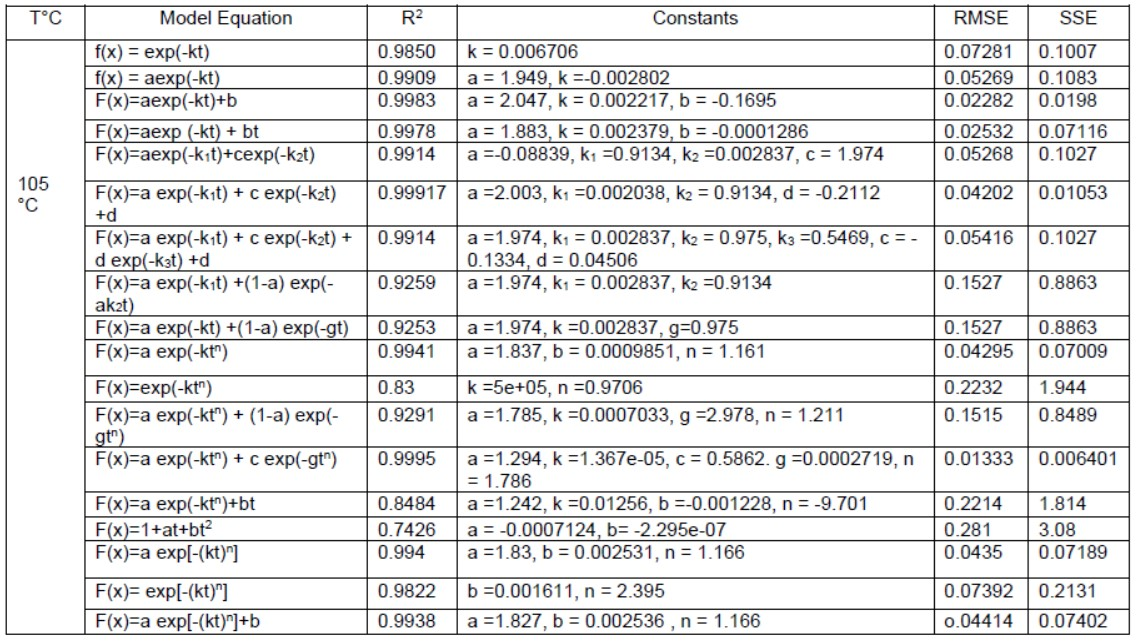Table 3. Statistical Results and Drying Constant of Mathematical Models at Different Temperature Drying (T=120 °C)